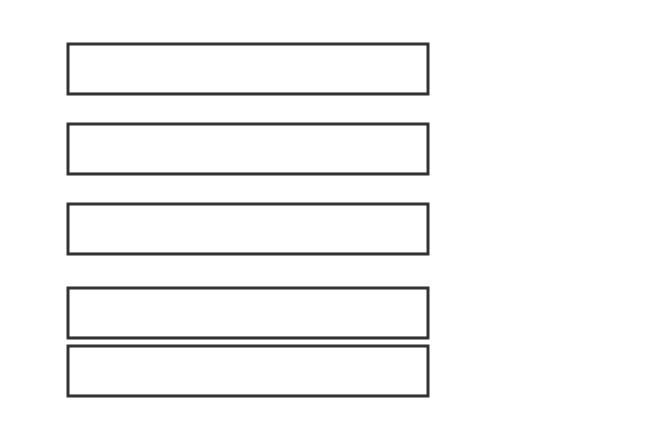# Like fractions

The two or more fractions that contain same denominator with different numerators are called the like fractions.

## Introduction

In some cases, if we observe two or more fractions, they may have same denominator and it seems they are similar. So, the fractions are called as the like fractions.

The like fractions are possibly formed if two or more quantities are divided as same number of parts. The division brings the property of similarity in the fractions.

### Example

The concept of like fractions can be understood from a geometrical example. Take five rectangles and split each rectangle into six equal parts but take two rectangles as a group.1. If we select two parts from first rectangle, then the fraction is equal to $\large \frac{2}{6}$
2. If we select three parts from second rectangle, then the fraction is equal to $\large \frac{3}{6}$
3. If we select five parts from third rectangle, then the fraction is equal to $\large \frac{5}{6}$
4. If we select ten parts from the remaining rectangles, then the fraction is equal to $\large \frac{10}{6}$

Thus, three proper fractions $\dfrac{2}{6}$, $\dfrac{3}{6}$ and $\dfrac{5}{6}$, and an improper fraction $\dfrac{10}{6}$ are formed. In this case, the denominator (consequent) is same though they are different fractions. Therefore, the fractions are called as like fractions.

Therefore, the like fractions can be either proper fractions or improper fractions or both.

###### Recommended
Latest Math Topics
Jun 26, 2023
Jun 23, 2023

Latest Math Problems
Jul 01, 2023
Jun 25, 2023
###### Math Questions

The math problems with solutions to learn how to solve a problem.

Learn solutions

Practice now

###### Math Videos

The math videos tutorials with visual graphics to learn every concept.

Watch now

###### Subscribe us

Get the latest math updates from the Math Doubts by subscribing us.